Courses

# Test: Data Handling - 2

## 10 Questions MCQ Test Mathematics (Maths) for Class 3 | Test: Data Handling - 2

Description
This mock test of Test: Data Handling - 2 for Class 3 helps you for every Class 3 entrance exam. This contains 10 Multiple Choice Questions for Class 3 Test: Data Handling - 2 (mcq) to study with solutions a complete question bank. The solved questions answers in this Test: Data Handling - 2 quiz give you a good mix of easy questions and tough questions. Class 3 students definitely take this Test: Data Handling - 2 exercise for a better result in the exam. You can find other Test: Data Handling - 2 extra questions, long questions & short questions for Class 3 on EduRev as well by searching above.
QUESTION: 1

###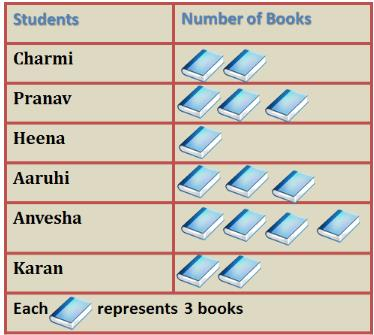How many more books did Anvesha read than Heena?

Solution:

Number of books read by each = number of picture of book x number of books represented by each picture

Number of books read by Heena =

1 x 3 = 3 books

Number of books read by Anvesha =

4 x 3 = 12 books

Difference = 12 - 3 = 9 books

QUESTION: 2

### DIRECTION: The given bar graph shows the amount of money spent by Amit in a week. Study the graph and answer the questions.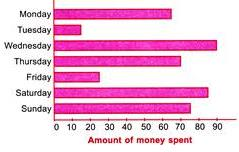Q. How much more amount Amit spent on Thursday than on Tuesday?

Solution:

Amount spent on Thursday = Rs. 70 Amount spent on Tuesday = Rs. 15 So, more amount spent on Thursday than on Tuesday = Rs. 70 − Rs. 15 = Rs. 55

QUESTION: 3

### DIRECTION: The given bar graph shows the amount of money spent by Amit in a week. Study the graph and answer the questions.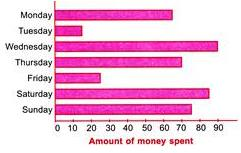Q. On which day Amit spent Rs. 65?

Solution:

According to the bar graph, on Monday Amit spent Rs. 65.

QUESTION: 4

DIRECTION: The given bar graph shows the amount of money spent by Amit in a week. Study the graph and answer the questions.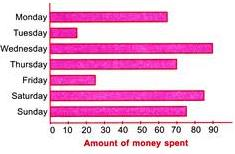Q. Find the amount of money spent by Amit on Sunday.

Solution:

Amount of money spent by Amit on Sunday = Rs. 75.

QUESTION: 5

DIRECTION: The given bar graph shows the amount of money spent by Amit in a week. Study the graph and answer the questions.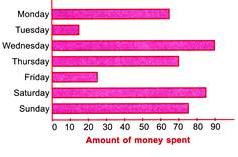Q. How much less Amount Amit spent on Friday than on Wednesday?

Solution:

Amount spent on Friday = Rs. 25 Amount spent on Wednesday = Rs. 90
∴ Required difference = Rs. 90 − Rs. 25 = Rs. 65. So, Amit spent Rs. 65 less on Friday than on Wednesday.

QUESTION: 6

DIRECTION: The given graph shows the number of people who attended a book fair. Study it carefully and answer the following questions.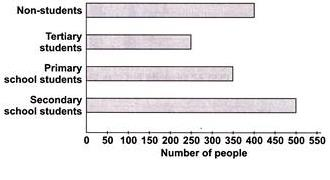Q. The number of secondary school students was twice the number of________.

Solution:

Number of secondary school students = 500
Number of tertiary students = 250
∴ Number of secondary school students = 2 × number of tertiary students

QUESTION: 7

DIRECTION: The given graph shows the number of people who attended a book fair. Study it carefully and answer the following questions.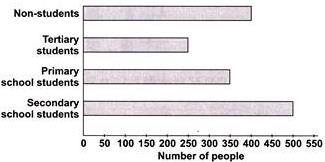Q. How many less tertiary students attended book fair than primary school students?

Solution:

Number of primary school students = 350 Number of tertiary students = 250
∴ Difference = 350 − 250 =100 So, 100 less tertiary students visited book fair than primary school students.

QUESTION: 8

DIRECTION: The given graph shows the number of people who attended a book fair. Study it carefully and answer the following questions.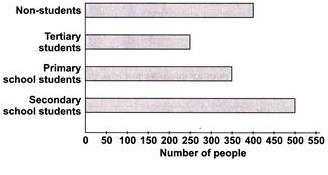Q. Number of secondary school students are ______ more than non-students.

Solution:

Number of secondary school students = 500 Number of non-students = 400
∴ Difference = 500 − 400 =100 So, 100 more secondary school students visited book fair than non-students.

QUESTION: 9

DIRECTION: The given pictograph shows the number of 4 different kinds of plants Mohit bought. Study the pictograph and answer the questions.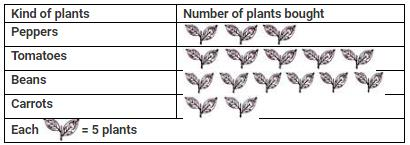Q. How many more tomato plants did he buy than pepper plants?

Solution:

Number of tomato plants he bought = 5 × 5 = 25
Number of pepper plants he bought = 3 × 5 = 15
∴ Difference = 25 − 15 =10 So, he bought 10 more tomato plants.

QUESTION: 10

DIRECTION: The given pictograph shows the number of 4 different kinds of plants Mohit bought. Study the pictograph and answer the questions.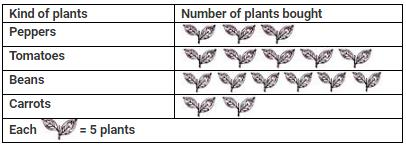Q. How many bean plants did he buy?

Solution:

Number of bean plants he bought = 6 × 5 = 30